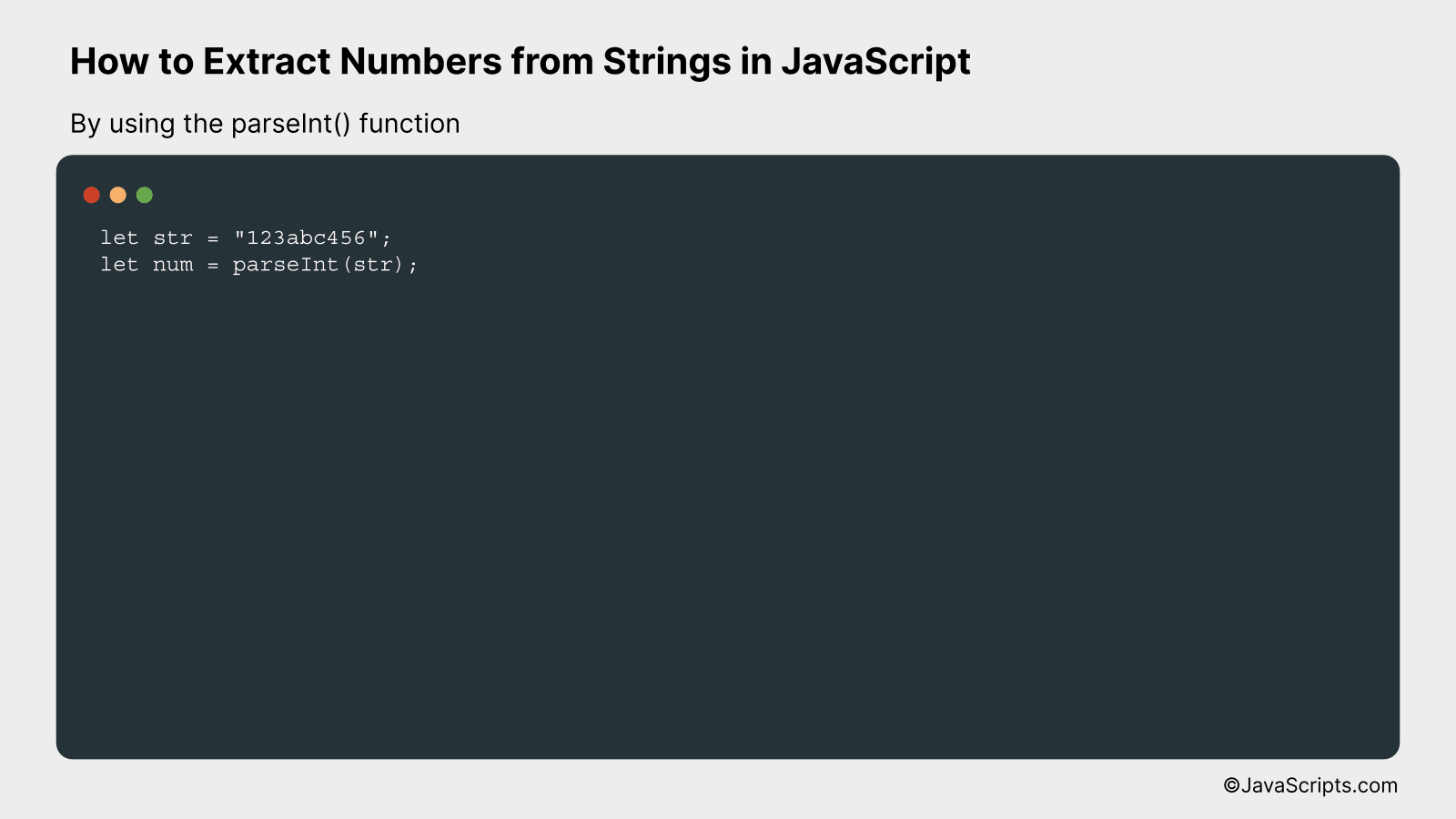# How to Extract Numbers from Strings in JavaScript

Welcome to a fascinating exploration of JavaScript! We all know that JavaScript, as a versatile scripting language, allows us to perform an array of tasks, from creating interactive web pages to handling data in complex ways. One such intriguing task we frequently encounter is extracting numbers from strings.

Now, you might ask, why is this important? Well, you and I regularly deal with data that comes as a mix of numbers and text, especially when working with user inputs or data feeds. Extracting numbers becomes essential to perform calculations or data manipulations. So, let’s dive into this together and discover simple techniques to accomplish this task effectively.

In this post, we will be looking at the following 3 ways to extract numbers from strings in JavaScript:

• By using the match() method with a Regular Expression
• By using the parseInt() function
• By using the parseFloat() function

Let’s explore each…

## #1 – By using the match() method with a Regular ExpressionIn JavaScript, we can use the match() method in combination with a Regular Expression (RegExp) to extract numbers from a string. This process involves searching the string for matches to the regular expression and returning an array of these matches.

``````
let str = "I have 2 apples and 3 oranges";
let numbers = str.match(/d+/g);
``````

### How it works

The match() method searches a string for a match against a regular expression, and returns the matches, as an Array object. In our example, we are using the regular expression /d+/g to find any number within the string.

• Step 1: The string “I have 2 apples and 3 oranges” is defined.
• Step 2: We apply the match() method to the string, passing it the regular expression /d+/g as a parameter.
• Step 3: The regular expression /d+/g targets any sequence of digits in the string. The d stands for digits, the + sign is a quantifier that means one or more, and the g is a flag indicating a global search, i.e., don’t stop at the first match but find all matches.
• Step 4: The match() method returns an array of all numbers found in the string.

## #2 – By using the parseInt() functionThe `parseInt()` function in JavaScript can extract the first number found in a string and convert it into an integer. For example, if we have a string “123abc456”, it will extract “123” and ignore the rest.

``````
let str = "123abc456";
let num = parseInt(str);
```
```

### How it works

The `parseInt()` function analyzes a string from left to right until it finds the first nonnumeric character, at which point it stops and returns the integer it has thus far.

• Step 1: The `parseInt()` function starts examining the given string from the first character.
• Step 2: It keeps scanning the string, character by character, as long as it keeps encountering digits (0 – 9).
• Step 3: As soon as it encounters a non-digit character, it stops scanning and returns the integer formed from the scanned digits.
• Step 4: The returned value is an integer version of the scanned part of the string. If the first character cannot be converted to a number, `parseInt()` returns NaN.

## #3 – By using the parseFloat() functionThe parseFloat() function in JavaScript takes a string as input and extracts the first valid floating point number from it, if one exists. For instance, if you have a string “123abc456”, parseFloat() will return 123 because this is the first valid number in the string.

``````
var str = "123abc456";
var num = parseFloat(str);
console.log(num); // Outputs: 123
``````

### How it works

The parseFloat() function starts scanning the string from the beginning and once it finds a character that can’t be part of a number (in our case, the letter ‘a’), it stops parsing and returns the already parsed number, or NaN if no number was detected.

Here are the specific steps:

• The function takes the string “123abc456” as an argument.
• Starting from the first character, it checks if it can be part of a valid number. It continues this process until hitting a character that can’t be part of a number (in our example, ‘a’).
• It then takes all the valid numeric (or decimal/minus sign) characters it has encountered up to that point and converts them into a floating-point number. In our example, this is the number 123.
• If the function didn’t find any characters that could be part of a number, it would return NaN.

Related:

In conclusion, mastering the process of extracting numbers from strings in JavaScript is a handy tool for any developer. It simplifies tasks by offering a straightforward way to manipulate and interact with numerical data embedded in strings.

Remember, the key methods involved are match() and regular expressions which make this process a breeze. So, keep practicing and soon you’ll find these methods become second nature to you in your programming journey.